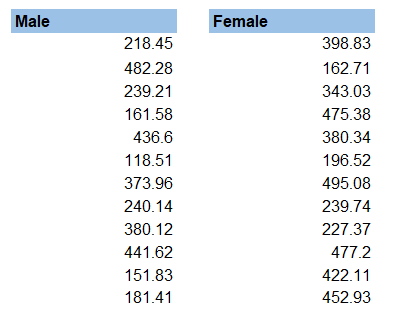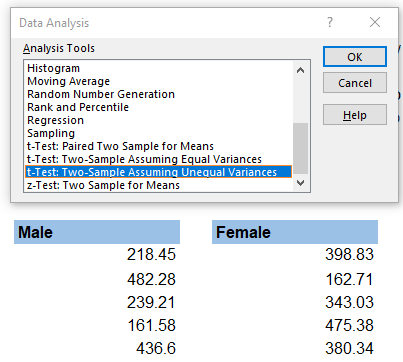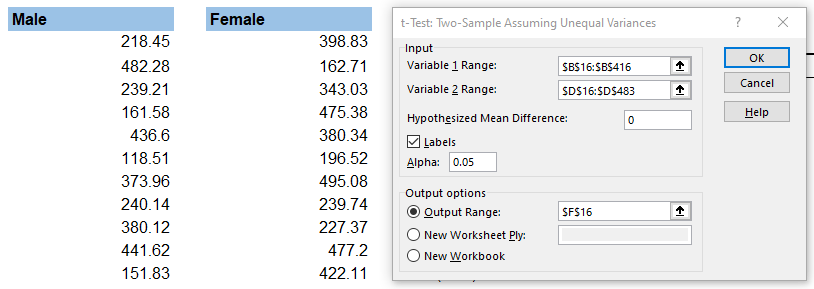## Hypothesis Testing for Complete Beginners

Gathering data in itself is meaningless unless we can analyze it and draw powerful insights. What makes data interesting is the ability to evaluate and interpret it.

Hypothesis testing refers to a term in statistics where we, as the analysts, evaluate an assumption related to a data set parameter.

Based on the purpose of the analysis and the specific characteristics of the data, we can use different methodologies. In general, the technique gives us a standardized way to assess the plausibility of an assumption based on sample data.

This sample data can originate either from a larger population or a data-generating process.

## What is a Hypothesis

Essentially, it is an educated guess, which we can test with observations or by experimenting. It can be anything, so long as it is testable.

When we propose a hypothesis, we write a hypothesis statement.

Generally, we strive to keep this in the form of ‘If… then…’. More specifically,

‘If A happens to an independent variable, then B will happen to the dependent variable.’

There are some characteristics to a well-written statement:

• As mentioned, it’s an if-then statement;
• We can test the statement scientifically;
• It states both the independent and dependent variables.

First, we define the problem we are analyzing, and then we base our hypothesis statement on this problem.

It is crucial to remember that the underlying assumption can be about any parameter of the population, and it can either be true or not.

## What is Hypothesis Testing

The best way to evaluate a hypothesis would be to review the entire population of the data we are analyzing. However, this usually proves to be highly impractical, if not wholly impossible. Therefore, we typically assess only a randomly selected sample instead of the entire population.

And if the data within the sample is not consistent with our hypothesis, we can reject it.

When we perform statistical analysis, we test a hypothesis by evaluating a random sample of the entire population. Practically, we test two hypotheses:

• The null hypothesis (H0)
• The alternative hypothesis (HA)

The null hypothesis usually assumes equality in parameters of the population, like the mean of the population is equal to zero. The alternative hypothesis then will be the exact opposite – the mean does not equal zero.

The null and alternative hypotheses have to be mutually exclusive. Only one can be correct, but one of the two is always right.The null hypothesis is usually the accepted fact – the mean equals zero, smoking causes cancer, loud music hurts your ears, and others. When we look at the randomly selected sample, we usually consider that the null hypothesis is that the observations are simply the result of chance. The alternative view is then that they are affected by a non-random cause.

## The Four Steps of Hypothesis Testing

We can present the process of data-driven decision making in four steps:

1. State the two hypotheses (null and alternative) in a way that only one can be true;
2. Plan how to evaluate the data and prepare the analysis plan, outlining how we will use the sample to assess the population. It is common to focus on a single parameter (e.g., mean, standard deviation, p-value, z-score, and others);
3. Evaluate the sample data and calculate the value of the test statistics, as described in the analysis plan;
4. Assess the results by applying the decision rules from the plan. Here we either accept the null hypothesis as plausible or reject it in favor of the alternative hypothesis

## Decision Rules

One of the most important things we need to define in our analysis plan is the set of decision rules for rejecting the null hypothesis, to be used in our assessment. In practice, these can be specified in two ways –referring to either a p-value or a region of acceptance.

A p-value measures the significance of the evidence in support of the null hypothesis. It is the probability of observing the test statistic with the assumption that the null is true. If the p-value is less than the significance level (our threshold), we reject the null hypothesis.

An acceptance region is a set of values for the test statistic. If it falls within those values, we fail to reject the null hypothesis. And values outside the region of acceptance fall within the region of rejection. If our test statistic ends up here, we reject the null. We can then say that we reject the null hypothesis at the α level of significance.

## Accepting or Failing to Reject

The testing has one of two outcomes – we accept the null hypothesis, or we reject it. However, most statisticians prefer to say they reject the null or fail to reject it instead of accepting it.

The idea behind is that saying we accept the null hypothesis means we deem it to be true while saying we fail to reject means we did not find the data to be persuasive enough to select the alternative over the null. Because we are performing a probabilistic test, there’s always a small chance of being wrong, and this different wording covers that.

## Errors

When we evaluate a hypothesis, we can end up with one of two types of errors:

Type I

This is when we reject the null hypothesis, but it is true. The probability of making a Type I error is the significance level, also called alpha (denoted α). In financial modeling and analysis, we would usually set alpha at 5% or 0.05. A smaller alpha (like 1%, or 0.1%) suggests a more robust evaluation of the null hypothesis.

Type II

We make this error when we fail to reject the null hypothesis, but it is false. The probability of making a Type II error is the beta, denoted β. On the other hand, the chance of not making such an error is called the power of the test.

## Interpreting the Results

We evaluate the p-value to portrait a finding as statistically significant by comparing the value of the statistical test to the predefined alpha level. If the p-value is less than the predefined threshold, then it has statistical significance.

From the perspective of hypothesis testing, if the p-value is less than (or equal to) the alpha, we reject the null hypothesis (significant result). If the p-value is higher than the alpha, we fail to reject the null (insignificant result).

The confidence level of the hypothesis for the observed data can be calculated as one minus alpha (1 – α). Knowing this, we have two ways to write up our conclusions.

• Fail to reject the null hypothesis at a 5% significance level; or
• Fail to reject the null hypothesis at a 95% confidence level.

When we interpret the p-value, it does not mean the null is true or false. It only means we have chosen to reject (or fail to reject) the null hypothesis at a specific confidence level based on the sample observations of the data. We cannot make binary decisions as we only rely on a probabilistic approach.

Critical Values

Instead of p-values, some tests may return a list of critical values, with their respective significance levels, and also a test statistic. We usually get such results in distribution-free hypothesis testing. However, the choice between p-value and critical values happens as part of the initial test design.

We similarly assess them by comparing the test statistic to the critical value at a chosen significance level. If the test statistic is higher than (or equal to) the critical value, we reject the null hypothesis. And conversely, if the test statistic is less than the critical value, we fail to reject the null.

We present the results in the same way as with p-values.

## Example

You can take a look at the following example to illustrate the concept better. The breakdown contains a sample of 1000 rows from our client’s online website sales. The extract has various information, but we will focus mainly on svar, showing us if the client was Male, Female, or not specified (empty cells) and the purchase value, pamount.The new sales director that our client hired is sure that statistically speaking, there’s no difference between male and female spending and believes the marketing targets should not reflect gender. However, our client has a long experience in the business and believes that men generally spend more on tech. This should be reflected by allocating a higher portion of the marketing budget towards advertising to men.

They have asked you to resolve their argument and suggest how to proceed.

Let’s start by writing our hypothesis statement:

If an online tech shopper is a man, then their purchase value will be different (higher).

We can then denote our null hypothesis as the difference in means for both observation sets (Male and Female) is equal to zero. And the alternative hypothesis will be the exact opposite – the difference in the means is not zero, meaning the average purchase values for both sets are statistically different.

First, we can separate our observations into two groups, as we will be comparing the mean (average) purchase value by Males versus Females.Now, let’s run the Data Analysis menu from the Data tab on the Ribbon:From the menu, select t-Test: Two-Sample Assuming Unequal Variances (as we don’t know the variances of the total populations):A dialog box opens up, where you will have to select the ranges for both variables (we now treat Males and Females as separate variables). Do not forget the check the Labels box if you select the headers of your data. The hypothesized mean difference will be zero, as per our null hypothesis.

In 99% of the cases, you can leave alpha at 0.05, as is, and you’ll be fine.Now run the analysis, and let’s look at the results.

The t-Test gives us some statistical information, but we are most interested in the two-tail p-value.But why do we pick two-tail and not one-tail? Well, our null hypothesis is that the difference in means is = to zero. Then if we reject the null, this means the difference can be either above zero or below zero. Because of this, we pick the two-tail p-value. The best way to fully understand this concept is to draw a simple chart with two ‘tails’ for the alternative hypothesis. The red areas form the rejection region, and the green arrow points to the single value that is our acceptance region.Therefore, we use the two-tail p-value of 0.038 and compare it to the alpha level of 0.05. The p-value is less than the level of significance (alpha), which means it is of statistical importance. We can reject the null hypothesis at the 95% confidence level. In conclusion, the data suggest that the difference between the average purchase value by males and females is significant. Therefore, it might be a good idea to do marketing separately.

Keep in mind the test result does not tell us if men spend more or less, just that there’s a statistically meaningful difference. Looking at our t-Test above, we see the mean for the Male variable is higher, which suggests men spend more on tech than women.

## Conclusion

Hypothesis testing is the process of evaluating a hypothesis either to reject it or to fail to reject it. It is important to remember that in this analysis, we rely on a probability-based approach, so we can never be 100% certain that our hypothesis is true or false. Coming up with a well-written hypothesis statement is one of the essential tasks of the process. It ensures that what we are testing relates to the problem we are analyzing.## FCCA, FMVA

Hi! I am a finance professional with 10+ years of experience in audit, controlling, reporting, financial analysis and modeling. I am excited to delve deep into specifics of various industries, where I can identify the best solutions for clients I work with.

In my spare time, I am into skiing, hiking and running. I am also active on Instagram and YouTube, where I try different ways to express my creative side.